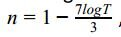# What fraction of light do these glasses transmit

• MHB
fxacx
Welders use a logarithmic scale to identify protective eyewear. The shade number n, is given by the
equation n = 1 − 7logT/3 , where T is the fraction of visible light that glass transmits.

a. What shade number should a welder use that only transmits ⅛ of the light entering the glass?
b Viewing a solar eclipse through #14 welding glasses is considered safe. What fraction of light do
these glasses transmit.

skeeter
Welders use a logarithmic scale to identify protective eyewear. The shade number n, is given by the
equation n = 1 − 7logT/3 , where T is the fraction of visible light that glass transmits.

a. What shade number should a welder use that only transmits ⅛ of the light entering the glass?
b Viewing a solar eclipse through #14 welding glasses is considered safe. What fraction of light do
these glasses transmit.

is the equation $n=1-7\log \left(\dfrac{T}{3}\right)$ ... ?

fxacx

#### Attachments

•yarab.JPG
1.5 KB · Views: 29
Homework Helper
Welders use a logarithmic scale to identify protective eyewear. The shade number n, is given by the
equation n = 1 − (7log(T))/3 , where T is the fraction of visible light that glass transmits.

a. What shade number should a welder use that only transmits ⅛ of the light entering the glass?
So T= 1/8. n= 1- (7 log(1/8))/3. Can you do the arithmetic?

b. Viewing a solar eclipse through #14 welding glasses is considered safe. What fraction of light do these glasses transmit.
Now n= 14 so 14= 1- (7 log(T))/3. 14- 1= 13= -(7/3) log(T).
-(3/7)(13)= -39/7= log(T). Do you know how to "reverse" log?

Last edited by a moderator: